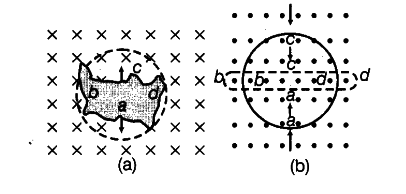# Use Lenz’s law to determine the direction of induced current in the situations described by figure

Use Lenz’s law to determine the direction of induced current in the situations described by figure.
(i) A wire of irregular shape turning into a circular shape.
(ii) A circular loop being deformed into a narrow straight wire.(i) Here, the direction of magnetic field is
perpendicularly inwards to the plane of paper. If a wire of irregular shape turns into a circular shape, then its area increases (v the circular loop has greater area than the loop of Irregular shape) so that, the magnetic flux linked also increases. Now, the induced current is produced in a direction such that it decreases the magnetic field [i.e. the current will flow in such a direction, so that the wire forming the loop is pulled inward in all directions (to decrease the area)], i.e. current is in anti-clockwise direction,